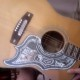## Last Year’s Final. Partly. Kind of.

I asked the 153 students to tell me a problem they’d like to see on the final exam (sort of as a challenge to other students)… the three that were named aloud today in the hearing of all present were p.806#41, p807#89, and p796#11.

I passed around last years exam and looked over quite a bit of that. To wit.

10. Find the derivative of the vector-valued function$\vec{r}(t) = \sec(t)\vec{i} + \tan(t)\vec{j}$.

The topic is barely introduced in this course; the whole message is “termwise differentiation works” (integration too of course… pesky constants emerge… you know the drill). That, and I get an excuse to bawl out the ones who botched “differentiate tan-x three times” in the middle of a Taylor Series calculation on the last exam.

9. Find the angle between$\langle - \sqrt{3}, 1\rangle$ and$\langle 3, \sqrt{3}\rangle$.

In the actual document I had the$\vec{i}, \vec{j}$ notation; in today’s whiteboard notes, I switched as I’ve done here. It looks cleaner and easier to understand to my eyes. Anyway, one has$\vec{v}\cdot\vec{w} = |\vec{v}| |\vec{w}| \cos(\theta)$, where theta is of course the desired angle; the “absolute value” is (again of course) the square root of the sum of the squares of the co-ordinates of the vector in question (its “norm”, “magnitude”, “length”, or what have you… ). These last two were “gift” problems offered by way of apology for not having had time to do much more than introduce certain topics. In the case of #10; it’s built into the syllabus… in place of #9 one would prefer a problem that uses the “angle” property in service of some geometric problem.

8. Find the equation of the plane through (2,2,0), (1,0,1), and (0,1,1).

I love this problem. Three points in (x,y,z)-space not all on a line; find a linear equation. The exact natural generalization of a problem we drill diligently into hundreds of Math 102 students every quarter [two points in (x,y)-space; find the linear equation]. That’s just got to be interesting. Sure enough, the picture, together with both products (“dot” and “cross”), tell the whole story; forget the messy “formula”.
See clearly how to do it and just do it this way.

Gotta go. More later.

Posted in 153. 1 Comment »

### One Response to “Last Year’s Final. Partly. Kind of.”

1.vlorbik Says:

“see clearly; … do it this way.”

or some other way; one can of course
set up three linear equations in x, y, and z
and solve by “103” methods.

i did this with the class just to keep
our feet on the ground. everybody gets it.